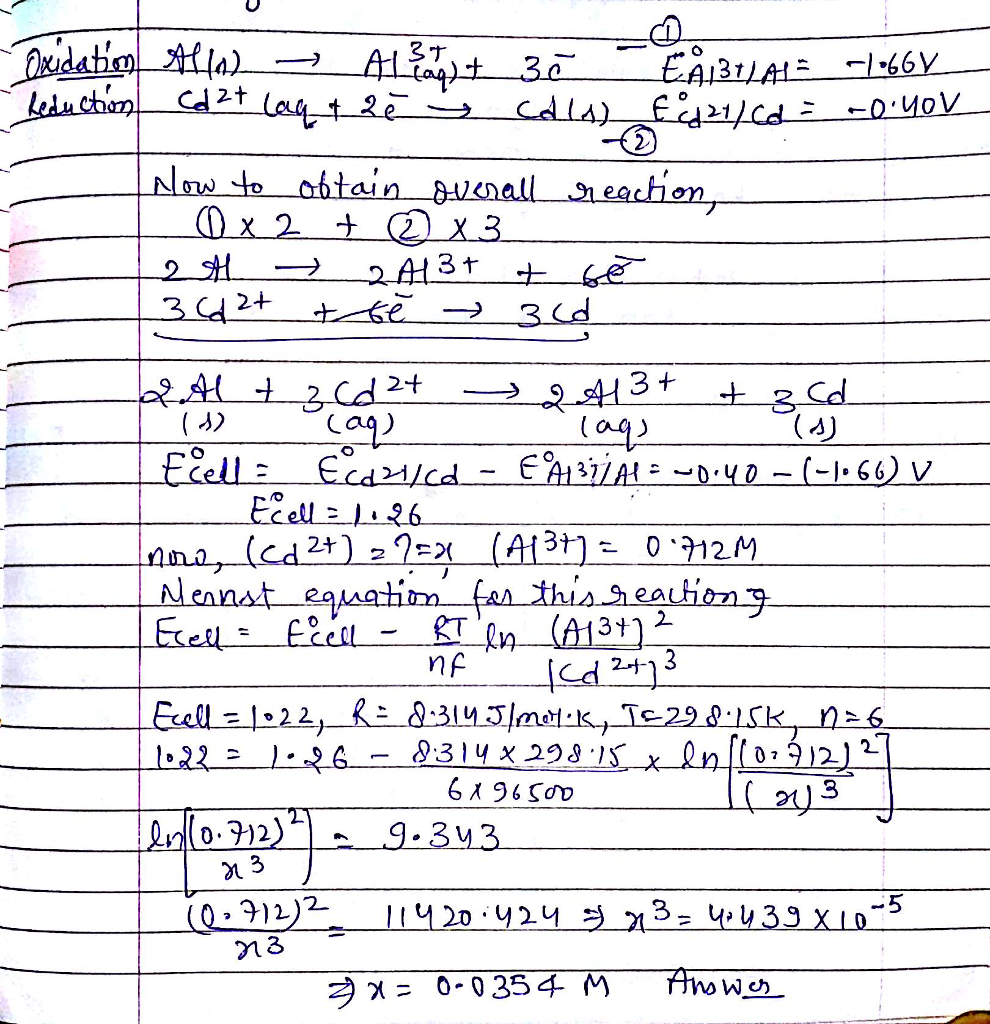# Question & Answer: A voltaic cell consists of an Al/Al3+ half-cell and a Cd/Cd2+ half-cell. Calculate {Cd2+} when…..

A voltaic cell consists of an Al/Al3+ half-cell and a Cd/Cd2+ half-cell. Calculate {Cd2+} when {Al3+} = 0.712 M and Ecell = 1.22 V. Use reduction potential values of Al3+ = -1.66 V and for Cd2+ = -0.40 V. (should be 0.035)